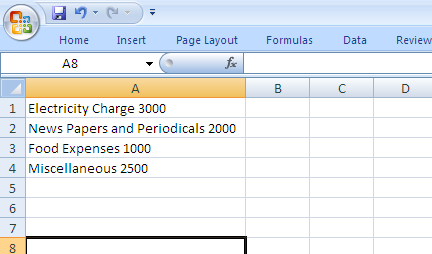## Thursday, 17 September 2015

### How to add User Defined Function in an Excel Work Book?

MS Excel is very powerful for processing worksheets and any data that can be organized in a tabular form. However  built-in function are not available  for some sort of problems. For example I can share a practical scenario for which one of my friends asked me a solution.

He has a file which contains multiple data records exported from some accounting software. Each record contains an amount as the last word of the line. Like follows

Electricity Charge 3000
News Papers and Periodicals 2000
Food Expenses 1000
Miscellaneous 2500

etc.

His requirement is quite simple. He want to calculate the total of these expenses. He copied this text to a Excel wok sheet. In Excel work sheet one line of text will be considered as the content of one cell. Like thisNow the amounts should be displayed in column B so that it can be processed as numeric values.

In excel function to extract last part of a text  is not available.

So I have added a new function in macro like below:

Function LASTWORD(strSting As String) As String
Dim strLastWord As String
Dim arrWords() As String

strSting = Trim(strSting)
arrWords = Split(strSting, " ")
strLastWord = arrWords(UBound(arrWords))

LASTWORD = strLastWord
End Function

Now you call this function from any cell as a normal excel function like below

=LASTWORD(C10)

## Tuesday, 6 January 2015

### Multiplication of two matrices / Matrix multiplication

Let us see how to multiply two matrices. Here is the rule applicable for the multiplication of two matrices. Multiplication of two matrices is possible only if the number of columns of the first matrix is equal to the number of rows of the second matrix.

For example it is possible to multiply two matrices with size  2 x 2 and 2 x 3. Here number of columns of first matrix ie. 2 is equal to the number of rows of the second matrix that is again  2.

Example:

C program for matrix multiplication

matmult.c

#include <stdio.h>
int main() {
int m, n, p, q, i, j, k, sum = 0;
int a, b, c;

clrscr();

// Input order or size of first matrix
printf("Enter the number of rows and columns of first matrix:");
scanf("%d%d", &m, &n);

// Input first matrix
for (i=0;i<m;i++)
for (j=0;j<n;j++) {
printf("Enter the element %d, %d of matrix a:", i,j);
scanf("%d", &a[i][j]);
}

// Input order or size of second matrix
printf("\nEnter the number of rows and columns of second matrix:");
scanf("%d%d", &p, &q);

// Check whether the matrices are compatible for multiplication
if (n!=p)
printf("\nMatrices with entered orders can't be multiplied with each other.");
else {
// Input second matrix
for (i=0;i<p;i++)
for (j=0;j<q;j++) {
printf("Enter the %d, %d element of  matrix b:", i, j);
scanf("%d", &b[i][j]);
}

// Logic for multiplication
for (i=0;i<m;i++) { // Loops row times of first matrix
for (j=0;j<q;j++) { // Loops column times of second  matrix
for (k=0;k<p;k++) { // Loops row times of  second matrix
sum=sum+a[i][k]*b[k][j];
}

c[i][j] = sum;
sum = 0;
}
}

// Displays first matrix
printf("Matrix A:\n");

for (i=0;i<m;i++) {
for (j=0;j<n;j++)
printf("%d\t", a[i][j]);
printf("\n");
}

// Displays second matrix
printf("Matrix B:\n");

for (i=0;i<p;i++) {
for (j=0;j<q;j++)
printf("%d\t", b[i][j]);
printf("\n");
}

// Displays third matrix
printf("Product of entered matrices:\n");

for (i=0;i<m;i++) {
for (j=0;j<q;j++)
printf("%d\t", c[i][j]);
printf("\n");
}
}

return 0;
}